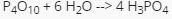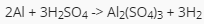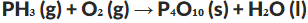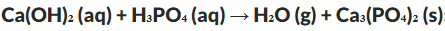# Stoichiometry and its Calculations

This set of MCQs helps you brush up on important chemistry topics and prepare you to dive into skill practice.

Start Quiz

If a chemist calculates the maximum amount of product that could be obtained in a chemical reaction, he or she is calculating the,

Percentage yield

Theoretical yield

Mole ratio

Actual yield

What is the measured amount of a product obtained from a chemical reaction?

Actual yield

Theoretical yield

Mole ratio

Percentage yield

What is the mole ratio of H2O to H3PO4 in the following chemical equation?2:3

1:3

6:4

4:6

How many grams are in 7.8 moles of NaCl?

450

346

476

452

How many grams of aluminum sulfate would be formed if 250g H2SO4 completely reacted with aluminum?120

430

290

80

When you balance,There are 4 moles of water produced per mole of P₄O₁₀.

There are 10 moles of water produced per mole of P₄O₁₀.

There are 1 moles of water produced per mole of P₄O₁₀.

There are 6 moles of water produced per mole of P₄O₁₀.

Balance :There are 1 moles of water produced per mole of calcium phosphate.

There are 6 moles of water produced per mole of calcium phosphate.

There are 2 moles of water produced per mole of calcium phosphate.

There are 10 moles of water produced per mole of calcium phosphate.

If these fertilizers are priced according to their nitrogen content, which will be the least expensive per 50 lb. bag?

Ammonia

Ammonium nitrate

Urea

Guanidine

Quiz/Test Summary
Title: Stoichiometry and its Calculations
Questions: 8
Contributed by: